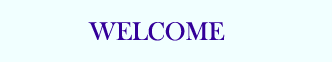TUTORIALS

C Quiz
C Exam

# Typecasting

Typecasting is a way to make a variable of one type, such as an int, act like another type, such as a char, for one single operation. To typecast something, simply put the type of variable you want the actual variable to act as inside parentheses in front of the actual variable. (char)a will make 'a' function as a char.

For example:
```#include <stdio.h>

int main()
{
/* The (char) is a typecast, telling the computer to interpret the 65 as a
character, not as a number.  It is going to give the character output of
the equivalent of the number 65 (It should be the letter A for ASCII).
Note that the %c below is the format code for printing a single character
*/
printf( "%c\n", (char)65 );
getchar();
}
```
One use for typecasting for is when you want to use the ASCII characters. For example, what if you want to create your own chart of all 128 ASCII characters. To do this, you will need to use to typecast to allow you to print out the integer as its character equivalent.
```#include <stdio.h>

int main()
{
for ( int x = 0; x < 128; x++ ) {
/* Note the use of the int version of x to output a number and the use
* of (char) to typecast the x into a character which outputs the
* ASCII character that corresponds to the current number
*/
printf( "%d = %c\n", x, (char)x );
}
getchar();

}
```
If you were paying careful attention, you might have noticed something kind of strange: when we passed the value of x to printf as a char, we'd already told the compiler that we intended the value to be treated as a character when we wrote the format string as %c. Since the char type is just a small integer, adding this typecast actually doesn't add any value!

So when would a typecast come in handy? One use of typecasts is to force the correct type of mathematical operation to take place. It turns out that in C (and other programming languages), the result of the division of integers is itself treated as an integer: for instance, 3/5 becomes 0! Why? Well, 3/5 is less than 1, and integer division ignores the remainder.

On the other hand, it turns out that division between floating point numbers, or even between one floating point number and an integer, is sufficient to keep the result as a floating point number. So if we were performing some kind of fancy division where we didn't want truncated values, we'd have to cast one of the variables to a floating point type. For instance, (float)3/5 comes out to .6, as you would expect!

When might this come up? It's often reasonable to store two values in integers. For instance, if you were tracking heart patients, you might have a function to compute their age in years and the number of heart times they'd come in for heart pain. One operation you might conceivably want to perform is to compute the number of times per year of life someone has come in to see their physician about heart pain. What would this look like?
```/* magical function returns the age in years */
int age = getAge();
/* magical function returns the number of visits */
int pain_visits = getVisits();

float visits_per_year = pain_visits / age;
```
The problem is that when this program is run, visits_per_year will be zero unless the patient had an awful lot of visits to the doc. The way to get around this problem is to cast one of the values being divided so it gets treated as a floating point number, which will cause the compiler to treat the expression as if it were to result in a floating point number:
```float visits_per_year = pain_visits / (float)age;
/* or */
float visits_per_year = (float)pain_visits / age;
```
This would cause the correct values to be stored in visits_per_year. Can you think of another solution to this problem (in this case)?